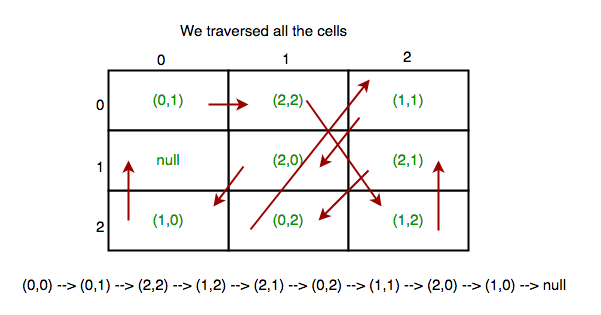Related Articles
Find if a 2-D array is completely traversed or not by following the cell values
• Difficulty Level : Easy
• Last Updated : 21 Aug, 2019

You are given a 2-D array. We have to traverse each and every cell of given array by following the cell locations then return true else false. The value of each cell is given by (x, y) where (x, y) is also shows next following cells position. Eg. (0, 0) shows starting cell. And ‘null’ shows our final destination after traversing every cell.Examples:

```Input : { 0, 1   1, 2   1, 1
0, 2   2, 0   2, 1
0, 0   1, 0   null }
Output : false

Input : { 0, 1   2, 0
null  1, 0
2, 1   1, 1 }
Output : true
```

## Recommended: Please try your approach on {IDE} first, before moving on to the solution.

We take a visited array if we visit a cell then make its value true in visited array, so that we can capture the cycle in our grid for next time when we visit it again. And if we find null before completing the loop then it means we didn’t traversed all the cell of given array.

## Java

 `/* Java program to Find a 2-D array is completely ` `traversed or not by following the cell values */` `import` `java.io.*; ` ` `  `class` `Cell { ` `    ``int` `x; ` `    ``int` `y; ` ` `  `    ``// Cell class constructor ` `    ``Cell(``int` `x, ``int` `y) ` `    ``{ ` `        ``this``.x = x; ` `        ``this``.y = y; ` `    ``} ` `} ` ` `  `public` `class` `MoveCellPerCellValue { ` ` `  `    ``// function which tells all cells are visited or not ` `    ``public` `boolean` `isAllCellTraversed(Cell grid[][]) ` `    ``{ ` `        ``boolean``[][] visited =  ` `              ``new` `boolean``[grid.length][grid[``0``].length]; ` ` `  `        ``int` `total = grid.length * grid[``0``].length; ` `        ``// starting cell values ` `        ``int` `startx = grid[``0``][``0``].x; ` `        ``int` `starty = grid[``0``][``0``].y; ` ` `  `        ``for` `(``int` `i = ``0``; i < total - ``2``; i++) { ` ` `  `            ``// if we get null before the end of loop  ` `            ``// then returns false. Because it means we  ` `            ``// didn't traverse all the cells ` `            ``if` `(grid[startx][starty] == ``null``)  ` `                ``return` `false``; ` `             `  `            ``// If found cycle then return false ` `            ``if` `(visited[startx][starty] == ``true``)  ` `                ``return` `false``; ` `             `  `            ``visited[startx][starty] = ``true``; ` `            ``int` `x = grid[startx][starty].x; ` `            ``int` `y = grid[startx][starty].y; ` ` `  `            ``// Update startx and starty values to next ` `            ``// cell values ` `            ``startx = x; ` `            ``starty = y; ` `        ``} ` ` `  `        ``// finally if we reach our goal then returns true ` `        ``if` `(grid[startx][starty] == ``null``)  ` `            ``return` `true``; ` `         `  `        ``return` `false``; ` `    ``} ` ` `  `    ``/* Driver program to test above function */` `    ``public` `static` `void` `main(String args[]) ` `    ``{ ` `        ``Cell cell[][] = ``new` `Cell[``3``][``2``]; ` `        ``cell[``0``][``0``] = ``new` `Cell(``0``, ``1``); ` `        ``cell[``0``][``1``] = ``new` `Cell(``2``, ``0``); ` `        ``cell[``1``][``0``] = ``null``; ` `        ``cell[``1``][``1``] = ``new` `Cell(``1``, ``0``); ` `        ``cell[``2``][``0``] = ``new` `Cell(``2``, ``1``); ` `        ``cell[``2``][``1``] = ``new` `Cell(``1``, ``1``); ` ` `  `        ``MoveCellPerCellValue mcp = ``new` `MoveCellPerCellValue(); ` `        ``System.out.println(mcp.isAllCellTraversed(cell)); ` `    ``} ` `} `

## C#

 `/* C# program to Find a 2-D array is completely ` `traversed or not by following the cell values */` `using` `System; ` ` `  `public` `class` `Cell ` `{ ` `    ``public` `int` `x; ` `    ``public` `int` `y; ` ` `  `    ``// Cell class constructor ` `    ``public` `Cell(``int` `x, ``int` `y) ` `    ``{ ` `        ``this``.x = x; ` `        ``this``.y = y; ` `    ``} ` `} ` ` `  `public` `class` `MoveCellPerCellValue  ` `{ ` ` `  `    ``// function which tells all cells are visited or not ` `    ``public` `Boolean isAllCellTraversed(Cell [,]grid) ` `    ``{ ` `        ``Boolean[,] visited =  ` `            ``new` `Boolean[grid.GetLength(0),  ` `                        ``grid.GetLength(1)]; ` ` `  `        ``int` `total = grid.GetLength(0) *  ` `                    ``grid.GetLength(1); ` `                     `  `        ``// starting cell values ` `        ``int` `startx = grid[0, 0].x; ` `        ``int` `starty = grid[0, 0].y; ` ` `  `        ``for` `(``int` `i = 0; i < total - 2; i++)  ` `        ``{ ` ` `  `            ``// if we get null before the end of loop  ` `            ``// then returns false. Because it means we  ` `            ``// didn't traverse all the cells ` `            ``if` `(grid[startx, starty] == ``null``)  ` `                ``return` `false``; ` `             `  `            ``// If found cycle then return false ` `            ``if` `(visited[startx, starty] == ``true``)  ` `                ``return` `false``; ` `             `  `            ``visited[startx, starty] = ``true``; ` `            ``int` `x = grid[startx, starty].x; ` `            ``int` `y = grid[startx, starty].y; ` ` `  `            ``// Update startx and starty values  ` `            ``// to next cell values ` `            ``startx = x; ` `            ``starty = y; ` `        ``} ` ` `  `        ``// finally if we reach our goal ` `        ``// then returns true ` `        ``if` `(grid[startx, starty] == ``null``)  ` `            ``return` `true``; ` `         `  `        ``return` `false``; ` `    ``} ` ` `  `    ``// Driver Code ` `    ``public` `static` `void` `Main(String []args) ` `    ``{ ` `        ``Cell [,]cell = ``new` `Cell[3, 2]; ` `        ``cell[0, 0] = ``new` `Cell(0, 1); ` `        ``cell[0, 1] = ``new` `Cell(2, 0); ` `        ``cell[1, 0] = ``null``; ` `        ``cell[1, 1] = ``new` `Cell(1, 0); ` `        ``cell[2, 0] = ``new` `Cell(2, 1); ` `        ``cell[2, 1] = ``new` `Cell(1, 1); ` ` `  `        ``MoveCellPerCellValue mcp = ``new` `MoveCellPerCellValue(); ` `        ``Console.WriteLine(mcp.isAllCellTraversed(cell)); ` `    ``} ` `} ` ` `  `// This code is contributed by Rajput-Ji `

Output:

```true
```

Time Complexity : O(N)
Auxiliary Space : O(M*N)

This article is contributed by Harshit Agrawal. If you like GeeksforGeeks and would like to contribute, you can also write an article using contribute.geeksforgeeks.org or mail your article to contribute@geeksforgeeks.org. See your article appearing on the GeeksforGeeks main page and help other Geeks.